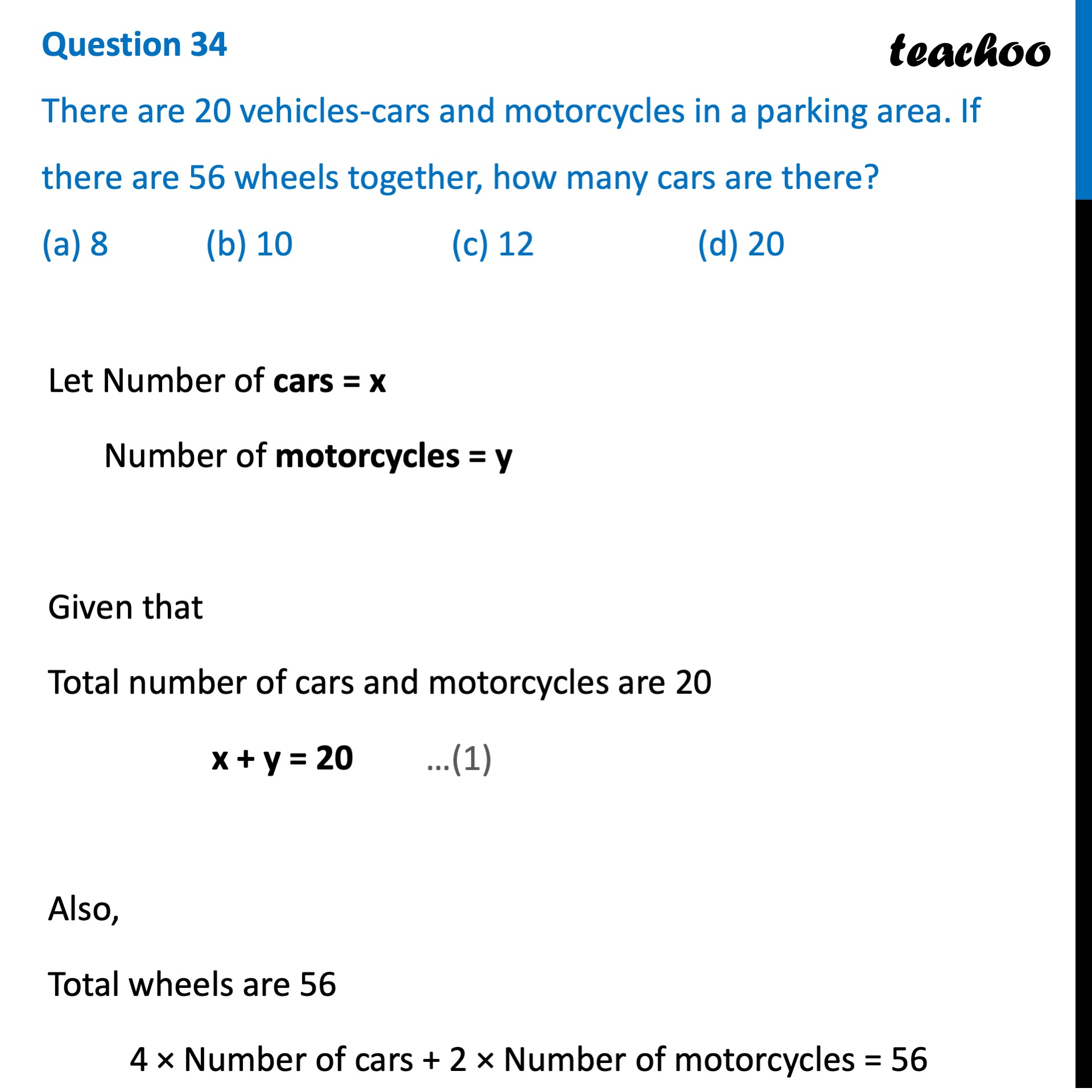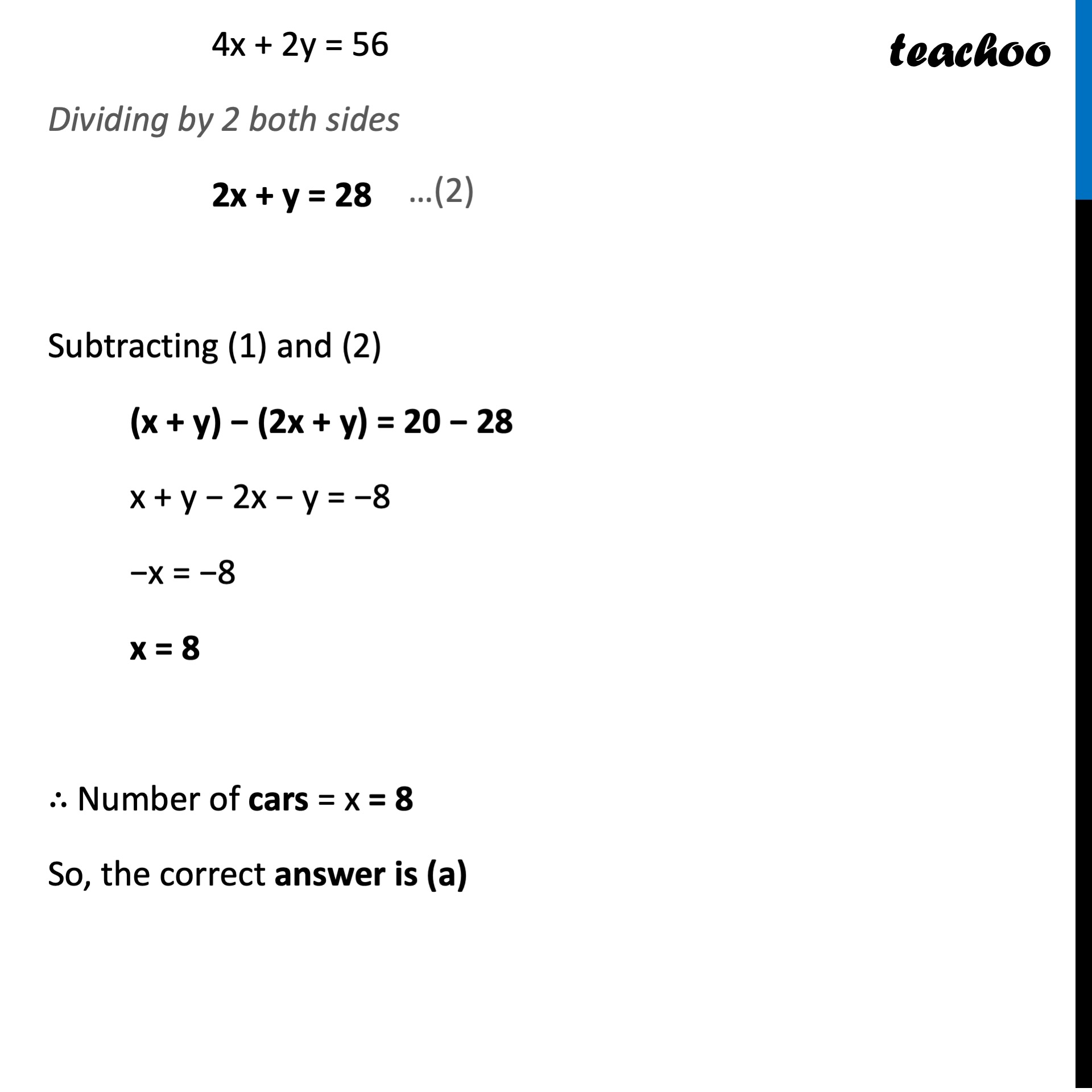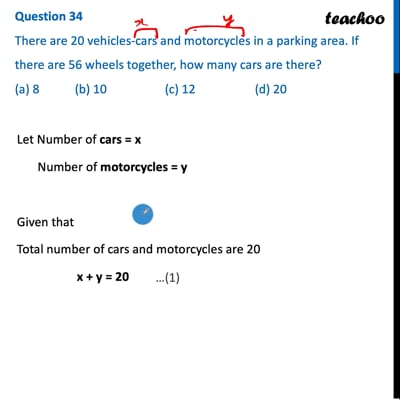CBSE Class 10 Sample Paper for 2022 Boards - Maths Basic [MCQ]

Class 10
Solutions of Sample Papers for Class 10 Boards

## (a) 8   (b) 10   (c) 12   (d) 20This video is only available for Teachoo black users

Introducing your new favourite teacher - Teachoo Black, at only ₹83 per month

### Transcript

Question 34 There are 20 vehicles-cars and motorcycles in a parking area. If there are 56 wheels together, how many cars are there? (a) 8 (b) 10 (c) 12 (d) 20 Let Number of cars = x Number of motorcycles = y Given that Total number of cars and motorcycles are 20 x + y = 20 Also, Total wheels are 56 4 × Number of cars + 2 × Number of motorcycles = 56 4x + 2y = 56 Dividing by 2 both sides 2x + y = 28 Subtracting (1) and (2) (x + y) − (2x + y) = 20 − 28 x + y − 2x − y = −8 −x = −8 x = 8 ∴ Number of cars = x = 8 So, the correct answer is (a)